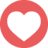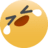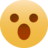# 約會巧遇高中家教女神，她給我的神回覆......

2018年12月5日 18:583582
．回應 136

cos(2x) = cos^2(x) - sin^2(x) = 2*cos^2(x) - 1 = 1 - 2*sin^2(x) tan(2x) = 2*sin(x)*cos(x) / (cos^2(x) - sin^2(x)) = 2*tan(x) / (1 - tan^2(x)) 就幫你到這了 === 因為三角函數上熱門到底是什麼妖術ಠ_ಠ

sin(2x)=2sinxcosx 幫你了 下面靠你自己了 我數學系～

cos(2x) = cos^2(x) - sin^2(x) = 2*cos^2(x) - 1 = 1 - 2*sin^2(x) tan(2x) = 2*sin(x)*cos(x) / (cos^2(x) - sin^2(x)) = 2*tan(x) / (1 - tan^2(x)) 就幫你到這了 === 因為三角函數上熱門到底是什麼妖術ಠ_ಠ

😂😂😂求後續

Ya

B15 學霸94狂 我要向您看齊

B17 可以嗎（星星眼

B17 我覺得在極座標下 r=k(1-sin(theta)) for k>0 應該可以啦嘻嘻 我是B15B15 文組人覺得神，完全看不懂。 （不過元po是高中家教，這是高中範圍嗎...？）